# TensorFlow从1到2（十五）（完结）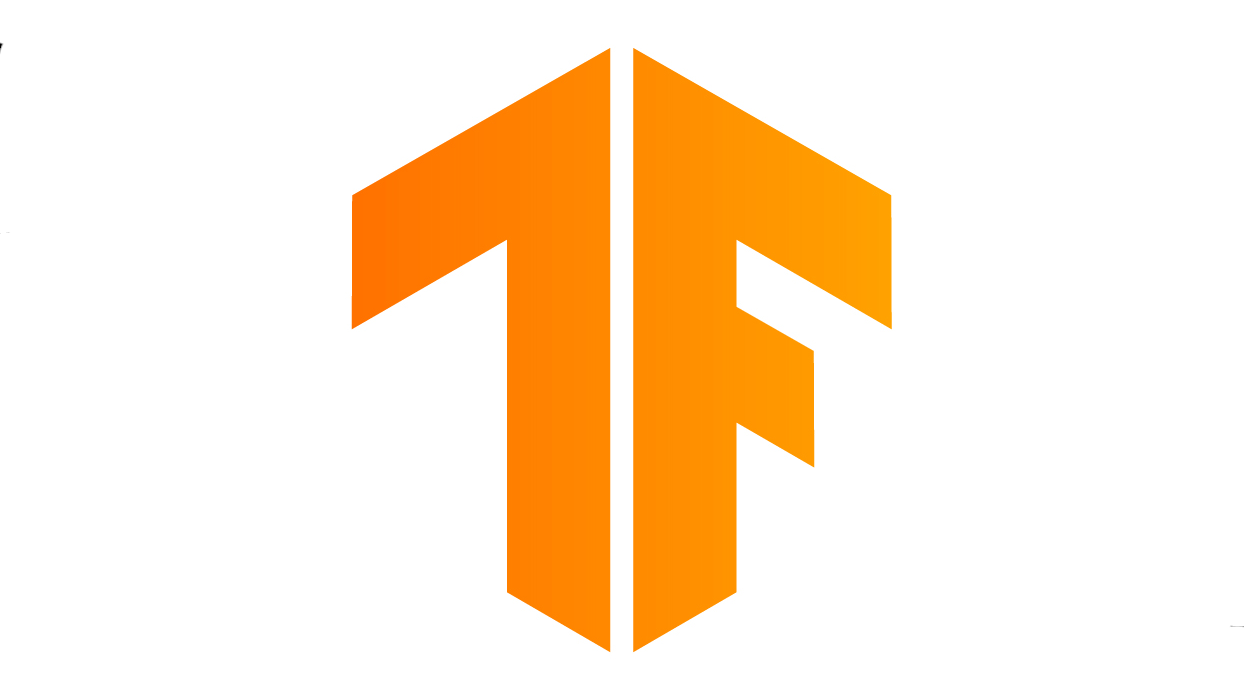#### TensorFlow的Javascript版

TensorFlow一直努力扩展自己的基础平台环境，除了熟悉的Python，当前的TensorFlow还实现了支持Javascript/C++/Java/Go/Swift(预发布版)共6种语言。

``````npm install @tensorflow/tfjs-node
# ...GPU版本...
npm install @tensorflow/tfjs-node-gpu
``````

``````npm install @tensorflow/tfjs
``````

#### 浏览器机器学习快速入门

``````<!DOCTYPE html>
<html>
<meta http-equiv="Content-Type" content="text/html; charset=utf-8">
<title>TensorFlow.js 练习</title>
<!-- 引入机器学习库TensorFlow.js -->
<script src="https://cdn.jsdelivr.net/npm/@tensorflow/tfjs@1.0.0/dist/tf.min.js"></script>
<!-- 引入机器学习可视化库tfjs-vis -->
<script src="https://cdn.jsdelivr.net/npm/@tensorflow/tfjs-vis@1.0.2/dist/tfjs-vis.umd.min.js"></script>
<!-- 机器学习主程序（自己编写） -->
<script src="script.js"></script>
<body>
<!-- 放你的网页内容 -->
本页无正文<p />
</body>
</html>
``````

``````[
{
"Name": "chevrolet chevelle malibu",
"Miles_per_Gallon": 18,
"Cylinders": 8,
"Displacement": 307,
"Horsepower": 130,
"Weight_in_lbs": 3504,
"Acceleration": 12,
"Year": "1970-01-01",
"Origin": "USA"
},
{
"Name": "buick skylark 320",
"Miles_per_Gallon": 15,
"Cylinders": 8,
"Displacement": 350,
"Horsepower": 165,
"Weight_in_lbs": 3693,
"Acceleration": 11.5,
"Year": "1970-01-01",
"Origin": "USA"
},
...
``````

``````// 获取数据，只保留感兴趣的字段，并进行数据清洗
async function getData() {
const carsData = await carsDataReq.json();
const cleaned = carsData.map(car => ({
//只保留两个字段
mpg: car.Miles_per_Gallon,
horsepower: car.Horsepower,
}))
//清洗无效数据
.filter(car => (car.mpg != null && car.horsepower != null));

return cleaned;
}
// 相当于主程序，执行入口
async function run() {
//载入数据
const data = await getData();
//建立绘图数据
const values = data.map(d => ({
x: d.horsepower,
y: d.mpg,
}));
//使用tfvis绘图
tfvis.render.scatterplot(
{name: 'Horsepower v MPG'},
{values},
{
xLabel: 'Horsepower',
yLabel: 'MPG',
height: 300
}
);
}
//载入后开始执行run()函数
``````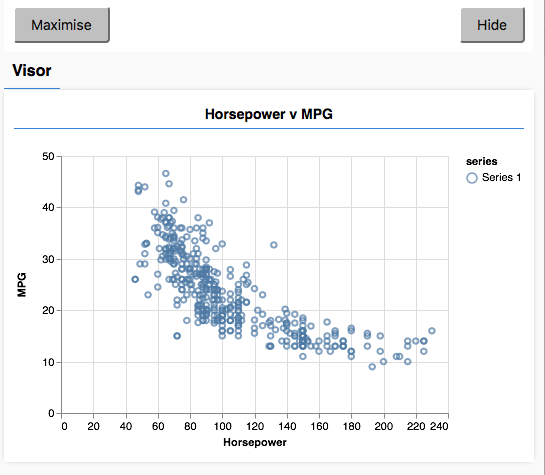#### 用js定义模型

TensorFlow.js完整模仿了Keras的模型定义方式，所以如果使用过Keras，那使用TensorFlow.js完全无压力。

``````// 建立神经网络模型
function createModel() {
// 使用sequential对象建立模型
const model = tf.sequential();
// 输入层
model.add(tf.layers.dense({inputShape: , units: 128, useBias: true}));
// 隐藏层
// 输出层

return model;
}
``````

``````// 在图表窗口显示模型摘要信息
tfvis.show.modelSummary({name: 'Model Summary'}, model);
``````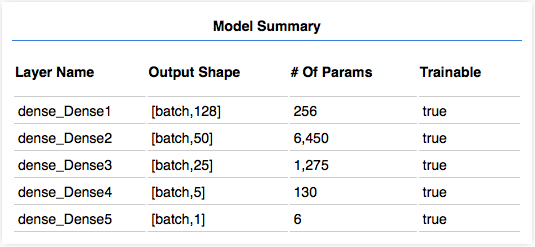#### 数据预处理

TensorFlow.js为了解决这个问题，专门提供了tf.tidy()函数。使用方法是把大规模的内存操作，放置在这个函数的回调中执行。函数调用完成后，tf.tidy()得到控制权，进行内存的清理工作，防止内存泄露。

``````// 将数据转换为张量
function convertToTensor(data) {
// 数据预处理的过程必然会产生很多中间结果，将占用大量内存
// tf.tidy()负责清理这些中间结果，所以要把数据处理包含在这个函数之内
// 这一点很重要
return tf.tidy(() => {
// 把样本数据乱序排列
tf.util.shuffle(data);

// 将数据转换为张量，功率值作为特征值，油耗值作为标定目标
const inputs = data.map(d => d.horsepower)
const labels = data.map(d => d.mpg);

const inputTensor = tf.tensor2d(inputs, [inputs.length, 1]);
const labelTensor = tf.tensor2d(labels, [labels.length, 1]);

// 数据规范化，把数据从最小到最大转换为0-1浮点空间
const inputMax = inputTensor.max();
const inputMin = inputTensor.min();
const labelMax = labelTensor.max();
const labelMin = labelTensor.min();

const normalizedInputs = inputTensor.sub(inputMin).div(inputMax.sub(inputMin));
const normalizedLabels = labelTensor.sub(labelMin).div(labelMax.sub(labelMin));

return {
inputs: normalizedInputs,
labels: normalizedLabels,
// 把数据范围值也要返回，我们后面绘图会用到
inputMax,
inputMin,
labelMax,
labelMin,
}
});
}
``````

#### 完整代码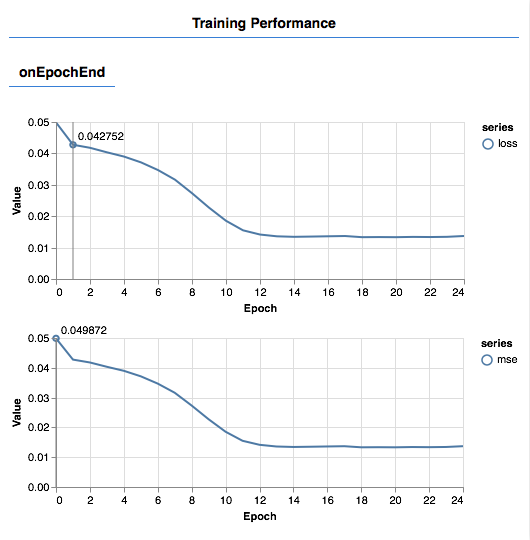``````// 获取数据，只保留感兴趣的字段，并进行数据清洗
async function getData() {
const carsData = await carsDataReq.json();
const cleaned = carsData.map(car => ({
mpg: car.Miles_per_Gallon,
horsepower: car.Horsepower,
}))
.filter(car => (car.mpg != null && car.horsepower != null));

return cleaned;
}
// 相当于主程序，执行入口
async function run() {
//载入数据
const data = await getData();
//建立绘图数据
const values = data.map(d => ({
x: d.horsepower,
y: d.mpg,
}));
//使用tfvis绘图
tfvis.render.scatterplot(
{name: 'Horsepower v MPG'},
{values},
{
xLabel: 'Horsepower',
yLabel: 'MPG',
height: 300
}
);
// 建立神经网络模型
const model = createModel();
// 在图表窗口显示模型摘要信息
tfvis.show.modelSummary({name: 'Model Summary'}, model);

// 将数据从js对象转换为张量，并完成预处理
const tensorData = convertToTensor(data);

// 使用样本数据训练模型，训练时只需要x/y的值
const {inputs, labels} = tensorData;
await trainModel(model, inputs, labels);
// 训练完成在console输出完成信息（需要打开浏览器console窗口才能看到）
console.log('Done Training');

// 使用训练完成的模型进行预测并显示结果
testModel(model, data, tensorData);
}

// 载入完成执行主函数run()

// 建立神经网络模型
function createModel() {
// 使用sequential对象建立模型
const model = tf.sequential();
// 输入层
model.add(tf.layers.dense({inputShape: , units: 128, useBias: true}));
// 隐藏层
// 输出层

return model;
}

// 将数据转换为张量
function convertToTensor(data) {
// 数据预处理的过程必然会产生很多中间结果，将占用大量内存
// tf.tidy()负责清理这些中间结果，所以要把数据处理包含在这个函数之内
// 这一点很重要
return tf.tidy(() => {
// 把样本数据乱序排列
tf.util.shuffle(data);

// 将数据转换为张量，功率值作为特征值，油耗值作为标定目标
const inputs = data.map(d => d.horsepower)
const labels = data.map(d => d.mpg);

const inputTensor = tf.tensor2d(inputs, [inputs.length, 1]);
const labelTensor = tf.tensor2d(labels, [labels.length, 1]);

// 数据规范化，把数据从最小到最大转换为0-1浮点空间
const inputMax = inputTensor.max();
const inputMin = inputTensor.min();
const labelMax = labelTensor.max();
const labelMin = labelTensor.min();

const normalizedInputs = inputTensor.sub(inputMin).div(inputMax.sub(inputMin));
const normalizedLabels = labelTensor.sub(labelMin).div(labelMax.sub(labelMin));

return {
inputs: normalizedInputs,
labels: normalizedLabels,
// 把数据范围值也要返回，我们后面绘图会用到
inputMax,
inputMin,
labelMax,
labelMin,
}
});
}
async function trainModel(model, inputs, labels) {
// 编译模型
model.compile({
loss: tf.losses.meanSquaredError,
metrics: ['mse'],
});
//每批次数据数量和训练迭代数量
const batchSize = 28;
const epochs = 25;

// 训练
return await model.fit(inputs, labels, {
batchSize,
epochs,
shuffle: true,
// 使用回调函数绘制训练过程，曲线指标loss/mse
callbacks: tfvis.show.fitCallbacks(
{ name: 'Training Performance' },
['loss', 'mse'],
{ height: 200, callbacks: ['onEpochEnd'] }
)
});
}

// 测试模型
function testModel(model, inputData, normalizationData) {
// 获取数据集的取值范围
const {inputMax, inputMin, labelMin, labelMax} = normalizationData;

// 防止内存泄露，依然要把大量数据的操作放在tf.tidy值中
const [xs, preds] = tf.tidy(() => {
//功率数据直接使用0-1空间，相当于遍历所有样本空间
const xs = tf.linspace(0, 1, 100);
//批量预测
const preds = model.predict(xs.reshape([100, 1]));

// 预测结果也是规范化的0-1值，所以使用数据集取值范围还原到原始样本模型
const unNormXs = xs
.mul(inputMax.sub(inputMin))
const unNormPreds = preds
.mul(labelMax.sub(labelMin))

// 返回最终结果
return [unNormXs.dataSync(), unNormPreds.dataSync()];
});
// 准备成绘图数据
const predictedPoints = Array.from(xs).map((val, i) => {
return {x: val, y: preds[i]}
});
// 原始的样本生成散列点同屏显示
const originalPoints = inputData.map(d => ({
x: d.horsepower, y: d.mpg,
}));

//绘图
tfvis.render.scatterplot(
{name: 'Model Predictions vs Original Data'},
{values: [originalPoints, predictedPoints], series: ['original', 'predicted']},
{
xLabel: 'Horsepower',
yLabel: 'MPG',
height: 300
}
);
}
``````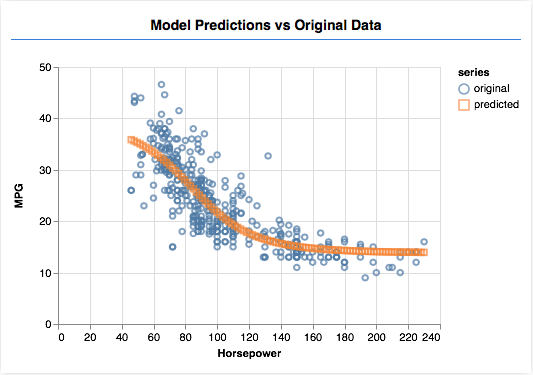#### 结语

（连载完）